# Test: Triangles- 2

## 25 Questions MCQ Test NCERT Mathematics for CAT Preparation | Test: Triangles- 2

Description
Attempt Test: Triangles- 2 | 25 questions in 25 minutes | Mock test for Class 9 preparation | Free important questions MCQ to study NCERT Mathematics for CAT Preparation for Class 9 Exam | Download free PDF with solutions
QUESTION: 1

### In ΔABC, AB = 2.5 cm and BC = 6 cm. Then, the length of AC cannot be

Solution:

in triangle ABC

AB = 2.5 cm

BC = 6 cm

AC =  ?

in any triangle sum of two sides >  third side

=> AB +  BC > AC

=> 2.5 + 6 > AC

=> AC < 8.5

AB + AC > BC

=> 2.5 + AC > 6

=> AC > 3.5

BC + AC > AB

=> 6 + AC > 2.5

=> AC > -3.5

Taking all together

3.5 < AC  < 8.5

3.6 ,  3.8 & 4  lies betwenn them

but not 3.4

Hence Length of AC can not be 3.4 cm

QUESTION: 2

Solution:
QUESTION: 3

### In the adjoining figure, △ABC ≅ △ADC. If ∠BAC = 30∘ and ∠ABC = 100∘ then ∠ACD is equal to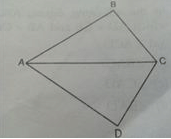Solution:
QUESTION: 4

It is not possible to construct a triangle when the lengths of its sides are

Solution:

For forming a triangle sum of any two sides must be greater than the third side.

QUESTION: 5

In fig, AC = BC and ∠ACY = 140. Find X and Y: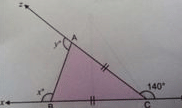Solution:
QUESTION: 6

In △ABC and △DEF, AB = DE and ∠A = ∠D.Then two triangles will be congruent by SA axiom if:

Solution:
QUESTION: 7

D is a Point on the Side BC of a △ABC such that AD bisects ∠BAC then:

Solution:
QUESTION: 8

In the adjoining figure, O is Mid – point of AB. If ∠ACO = ∠BDO, then ∠OAC is equal to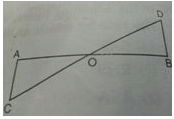Solution:
QUESTION: 9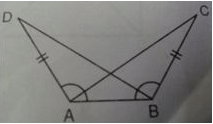Solution:
QUESTION: 10

In fig. which of the following statement is true?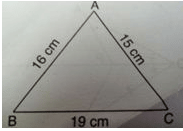Solution:
QUESTION: 11

In △ABC, ∠A = 35 and ∠B = 65, then the longest side of the triangle is:

Solution:
QUESTION: 12

In △ABC, if ∠A = 45 and ∠B = 70, then the shortest and the longest sides of the triangle are respectively,

Solution:
QUESTION: 13

In the adjoining figure, AB = BC and ∠ABD = ∠CBD, then another angle which measures 30 is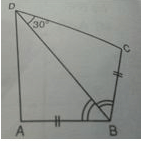Solution:

The two triangles are congruent by SAS congruency.

QUESTION: 14

ABC ≅ △PQR. If AB=5 cm, and then which of the following is true?

Solution:
QUESTION: 15

In fig., △ABD ≅ △ACD, AB = AC, name the criteria by which the triangles are congruent: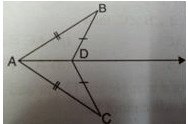Solution:
QUESTION: 16

P is a point on side BC of a △ABC such that AP bisects △BAC. Then

Solution:
QUESTION: 17

In the adjoining figure, AB = AC and ∠A = 70, then ∠C is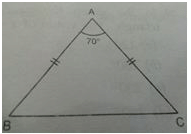Solution:
QUESTION: 18

In the adjoining figure, AC = BD. If ∠CAB = ∠DBA, then ∠ACB is equal to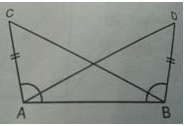Solution:
QUESTION: 19

In △ABC, if ∠B = 30 and ∠C = 70, then which of the following is the longest side?

Solution:
QUESTION: 20

In fig, in △ABD, AB = AC, then the value of x is: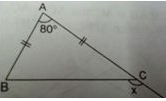Solution:
QUESTION: 21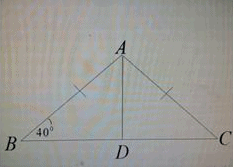Solution:
QUESTION: 22

In the adjoining fig, PQ = PR. If ∠QPR = 48, then value of x is: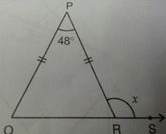Solution:
QUESTION: 23

In the adjoining figure, ABCD is a quadrilateral in which AD = CB and AB = CD, then ∠ACB is equal to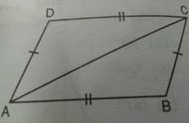Solution:
QUESTION: 24

In the adjoining figure, PQ > PR. If OQ and OR are bisectors of ∠Q and ∠R respectively, then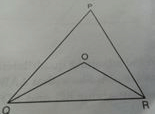Solution:
QUESTION: 25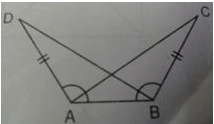Use Code STAYHOME200 and get INR 200 additional OFF Use Coupon Code